Question

Ansys

Given that E = 16,000 ksi, A = 6 in². Develop a finite-element model in ANSYS™ using top-down modeling in the following steps. Show screen captures of your work. Make sure you use a consistent set of units.

(d) Show a screen capture of your material properties and real constants

(b) Calculate the stress in element 1?

(g) Determine the axial-stresses in all the members? Create an output table with the axial stress values.

(a) Calculate the reaction forces?

(a) Create model geometry using the geometry for key points and lines indicated in the figure above. Present a screen capture of your model.

(e) Using the ANSYS™™ Post-processor, determine reaction forces? Show the output from ANSYSTM.

(f) Determine the nodal deflections for all the nodes? Create an output table with the nodal deflection values.

(c) Show a screen-capture of your meshed model with the applied loads and boundary conditions.

(b) Select an element type and justify your choice? Mesh the model such that is only one-element per line.Verified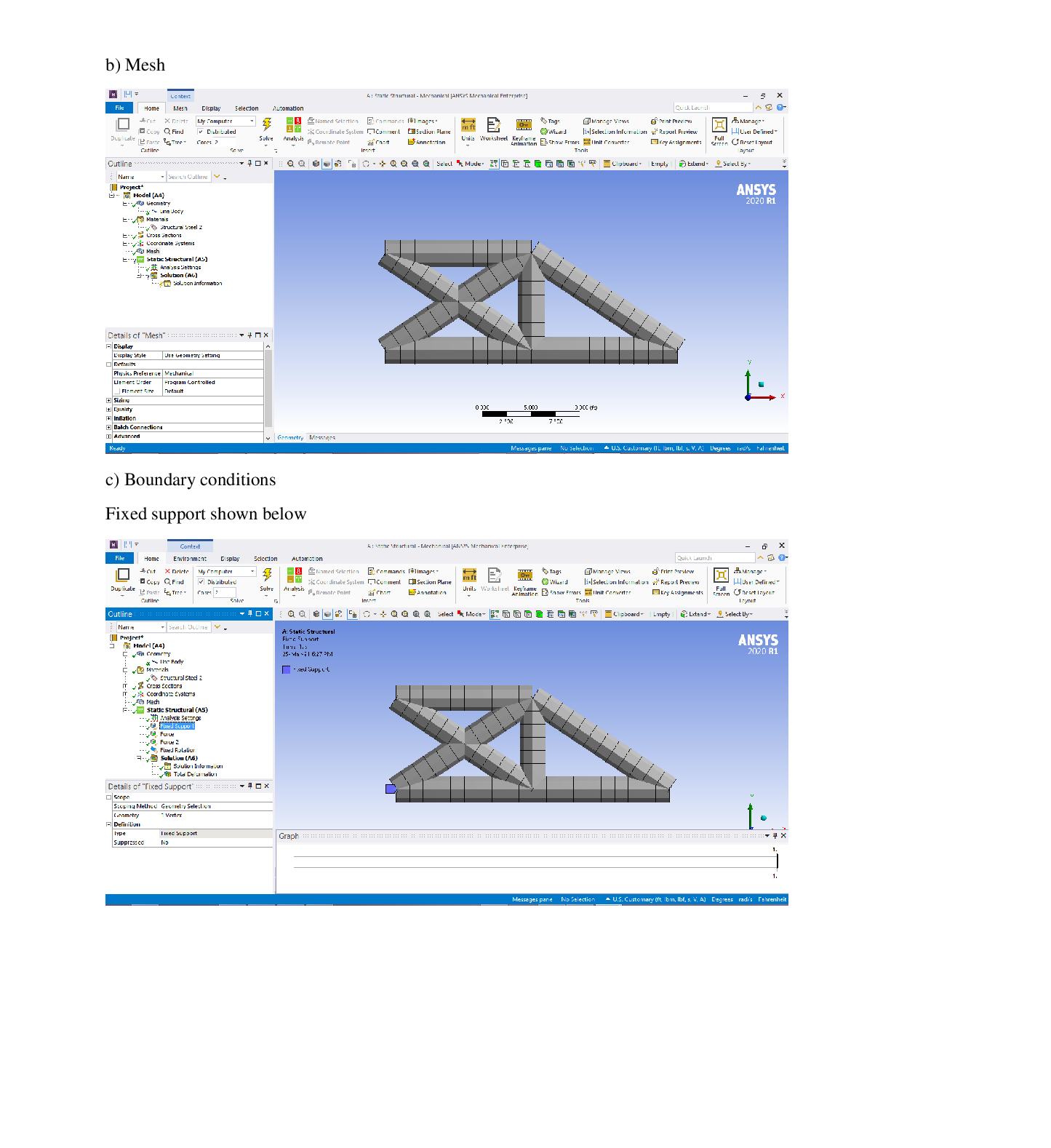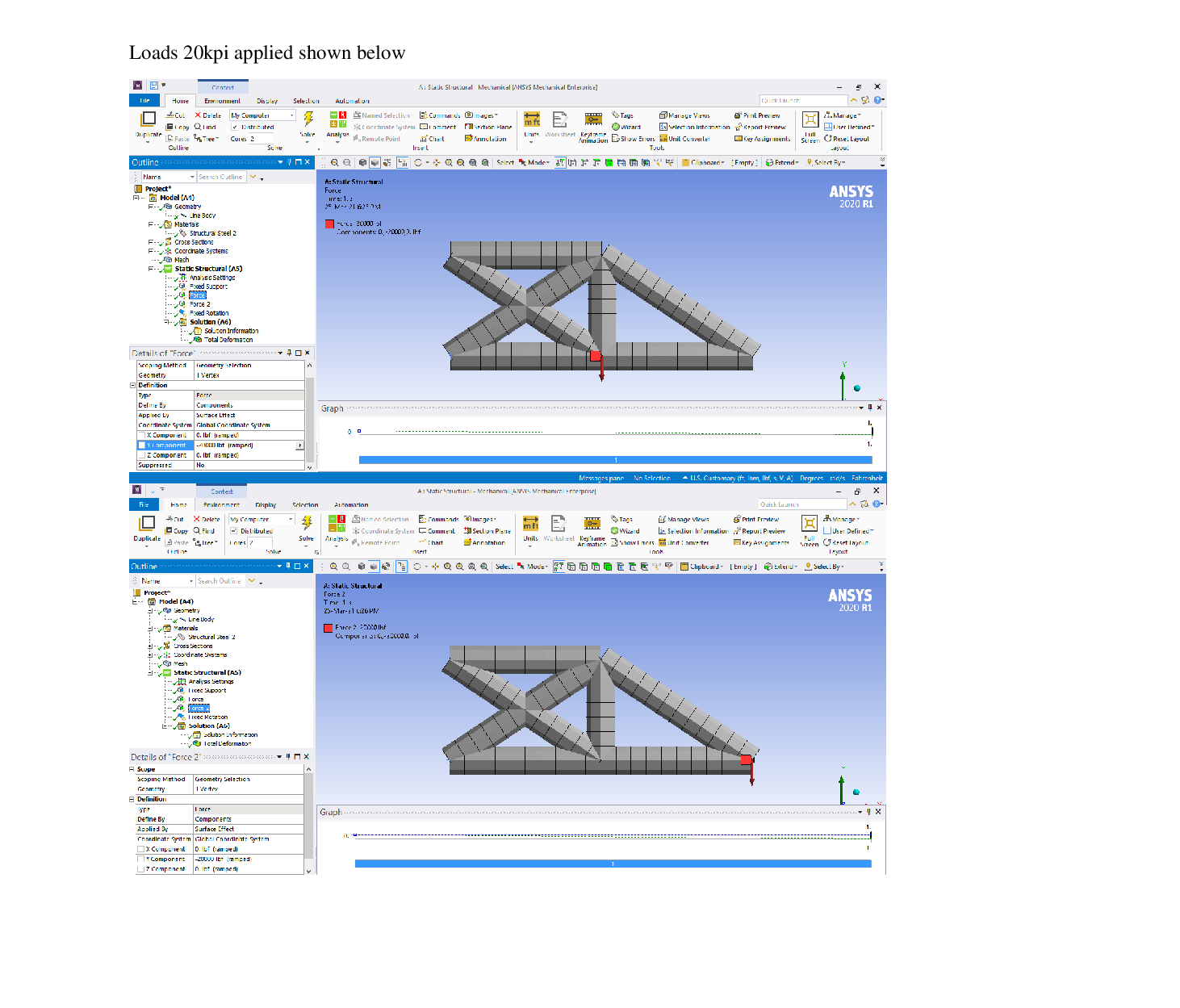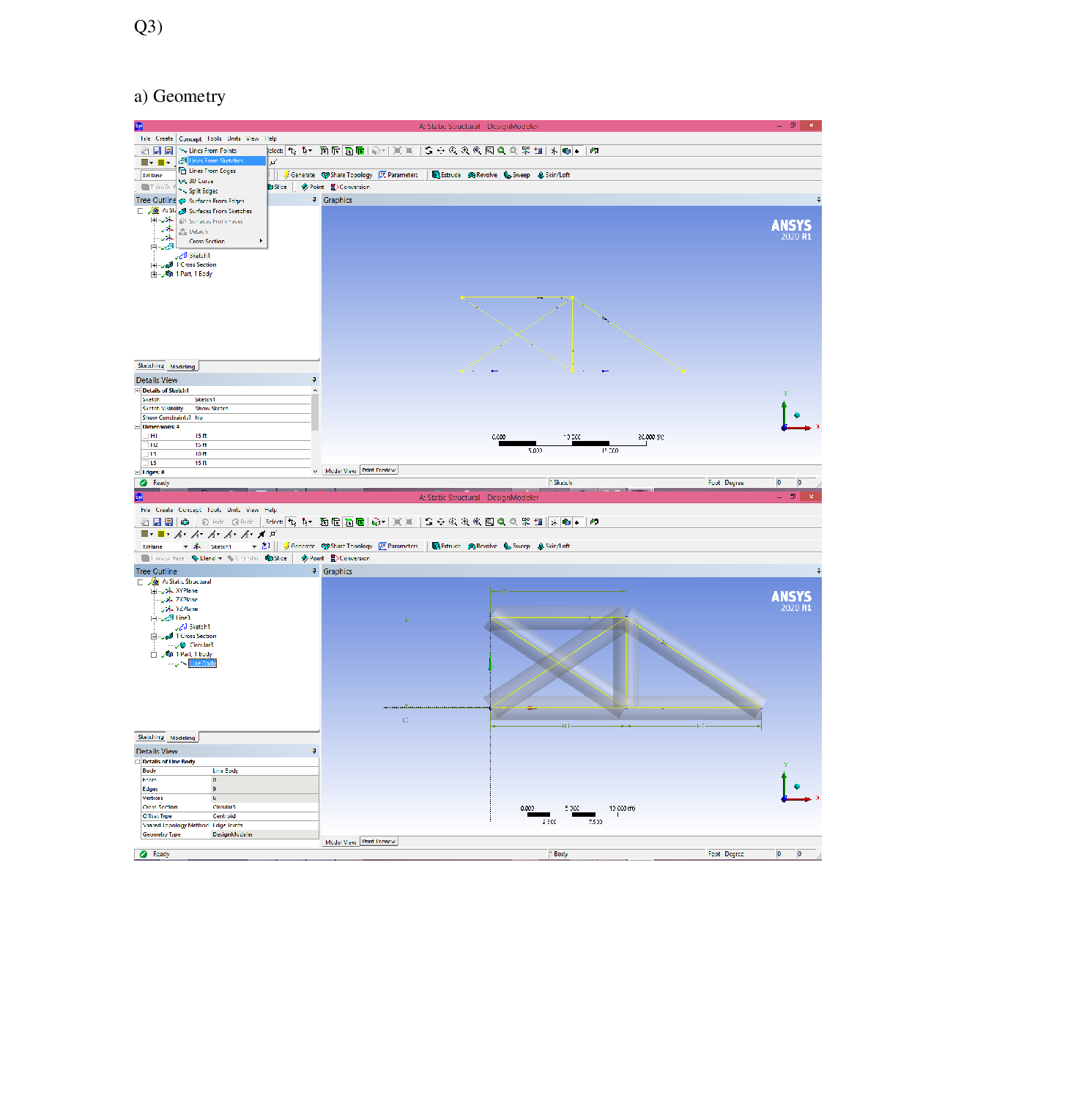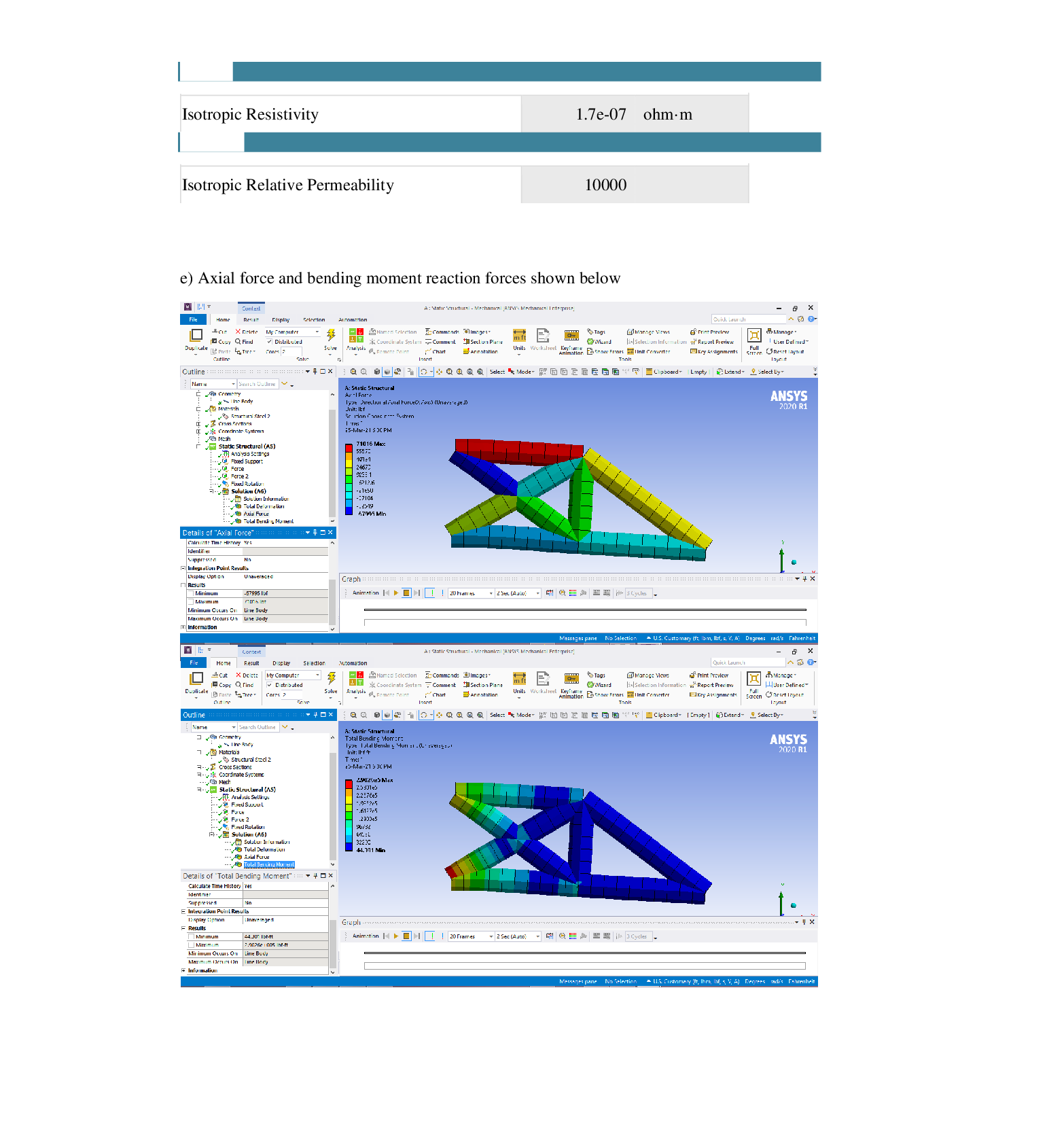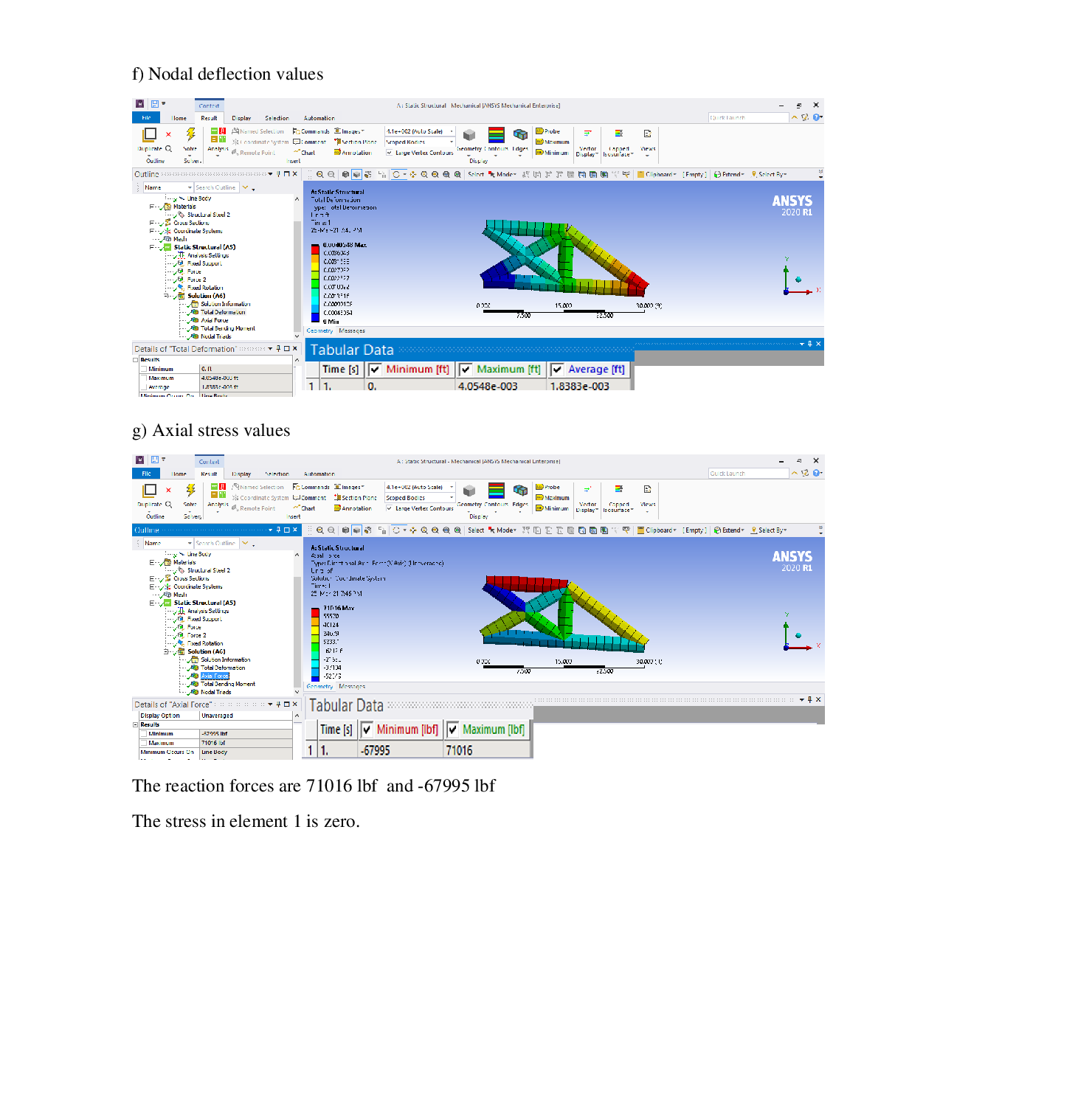### Question 33231Ansys

Given that E = 16,000 ksi, A = 6 in². Develop a finite-element model in ANSYS™ using top-down modeling in the following steps. Show screen captures of your work. Make sure you use a consistent set of units.
(b) Select an element type and justify your choice? Mesh the model such that is only one-element per line.
(e) Using the ANSYS™™ Post-processor, determine reaction forces? Show the output from ANSYSTM.
(f) Determine the nodal deflections for all the nodes? Create an output table with the nodal deflection values.
(c) Show a screen-capture of your meshed model with the applied loads and boundary conditions.
(d) Show a screen capture of your material properties and real constants
(b) Calculate the stress in element 1?
(g) Determine the axial-stresses in all the members? Create an output table with the axial stress values.
(a) Calculate the reaction forces?
(a) Create model geometry using the geometry for key points and lines indicated in the figure above. Present a screen capture of your model.

### Question 33230Ansys

A retractable stability-boom called the "outrigger", is attached to the back of a heavy-lift truck and is designed to provide stability for lifting heavy off-centered loads. Figure 1 shows the stability-boom attached to the back of a truck in its retracted position. Figure 2 shows the stability boom fully extended and engaged for an off-center lift.
Given that the stability-boom is a 3-stage telescopic arm as shown in Figure 2, made of structuralA-36 steel with the Elastic Modulus, E = 208 GPa, Poisson's Ratio, v = 0.33, and yield strength,Oys = 265 MPa. Assume that the 2-largest telescopic stages are rectangular-tubular in x-section and the final stage is a rectangular tube with closed end (Figure 2).
The stability boom is 1.75-meter long in its retracted position and 3.75-meter long in the fully extended configuration. Assume that each of the 3-telescopic stages have equal exposed lengths in the fully extended configuration.
The truck is designed for a load capacity of 60,845 kg on the free-tip of the stability boom.Determine the x-section dimensions of the stability boom using the following steps:
(e) Show the contour plot of the von-mises stress, and the longitudinal normal-stress along the length. Identify the location of maximum stress in the stability boom?
(f) Using your finite element model, show if a higher load capacity can be attained using an alternate cross-section of the stability boom? Show all the sections analyzed.
(c) Determine the required section dimensions for the stability-boom iteratively starting from your initial guess in Part (b), using both the BEAM model and the Solid Model? Assume a Factor-of-Safety of 3 at maximum load. Show all the cases analyzed. Clearly list any section-properties used.
(d) Determine the free-end deflection at maximum load? Show the deformed and undeformed configuration of the stability-boom.
(a) Develop a Conceptual-Model for the fully-extended configuration of the stability-boom.Identify any assumptions, constraints, and boundary conditions. Justify why they are reasonable, and why the conceptual-model of the physical structure will represent the structure accurately.
(b) Based on the Conceptual-Model in Part (a), develop a Finite-Element Model of the stability boom using (i) BEAM Elements (ii) Solid Elements. Initially assume the cross-section dimensions.Show screen-shots of your meshed-model with dimensions, boundary conditions, and loads.

### Question 33229Ansys

A simple bracket shown in the following figure is built from a 30 mm thick steel plate. This plate is fixed at the two small holes on the left and has a load applied to the larger hole on the right.Given that E =208GPA, and v = 0.33.
(a) Create the geometry in ANSYS™ and mesh the model. Show screen captures of meshed model with applied BCs.
(b) Calculate the peak displacement .at the right end of the model? Show screen captures of dof contour joints
(c) What type of elements did you use? Why? What assumptions if any have you made in the model development? Justify assumptions.
) Has your solution converged? Justify?
(e) Calculate the relative error in your model?

### Question 24950Ansys

Being a consultant for the above project, you have also been asked to assist in reducing the NOx emissions of the above cycle. Can you recommend methods to reduce NOx emissions and give their details?
[2 points](d) Explain the detailed operation of the Atkinson cycle, and explain why it has been used for many hybrid electric vehicles. Why does the Atkinson cycle have a higher efficiency than the Otto cycle?[2.5 points]
(e) In a Rankine cycle, why is it worth bleeding off some of the steam before the HP turbine to preheat the steam entering the boiler?[1.5 points]
(c) If the aim is to reduce CO2 emissions from a car, would you select an engine operating on an Otto cycle or a Diesel cycle? Discuss your selection.
(b) Explain the changes required for using an air conditioning system for a room as a refrigeration system for the storage of frozen food.[2.5 points]
(a) Why NOx emissions are higher in diesel engines?

### Question 24948Ansys

The Brayton cycle shown in Figure 1 has reheat and two compressors with a flow rate of 210 kg s-1 of air. Assume this system is being used in Tuscaloosa, AL. The inlet conditions to the first compressor is 1 bar and the inlet temperature would be current temperature of Tuscaloosa in °C (to the nearest whole number value, check current temperature and use it). Both compressors have a pressure ratio of 5:1. The inlet temperature for the second compressor is 40°C. The inlet temperature of first turbine is 970°C. The first turbine drops the pressure to 5 bar and then the air is reheated to927°C before it enters the reheat turbine. There is no pressure drop across the heat exchangers. The approach temperature for the heat exchanger (between state 9 and state 10, as shown in Figure 1) will be last two digits of your CWID (eg. If your CWIDis 12387854, then approach temperature will be 54°C). The isentropic efficiency of both turbines is 92% and that of the compressors is 88%. Use y (ratio of the specific heats) = 1.4 and Cp (specific heat at constant pressure) = 1.005 kJ kg-1 K-1.%3D
What is the thermal efficiency of the cycle?

### Question 11151Ansys

Describe the resulting velocity and temperature distribution at the outlet of the computational domain, for the case of Uhot water=D1.2 m/s,

### Question 11150Ansys

Describe the behaviour of the residuals.

### Question 11149Ansys

What is the thickness of the boundary layer near the solid walls? How did you calculate it?

### Question 11148Ansys

Briefly describe the boundary conditions and the fluid properties in your CFD simulations.
For the toolbar, press ALT+F10 (PC) or ALT+FN+F10 (Mac),

### Question 8588Ansys

22. In Problem 21, indicate the value in register A.

### Submit query